# Filtering

#### Introduction

Filtering is a technique for modifying or enhancing an image. For example, you can filter an image to emphasize certain features or remove other features. Filtering is a neighborhood operation, in which the value of any given pixel in the output image is determined by applying some algorithm to the values of the pixels in the neighborhood of the corresponding input pixel. A pixel’s neighborhood is some set of pixels, defined by their locations relative to that pixel.

#### Linear & Non-Linear

Linear Filtering occurs when the operation performed on each pixel is a  simple matematical operation with a scalar where the result is similar for all pixels. For example, if we are multiplying the intensity of each pixel by 2, then the entire image gets intensified by a factor of two which means we have effectively multiplied the image matrix by 2. On the other hand in a non-linear operation, the overall effect on the image cannot be predicted just by the operation performed on each pixel. For example squaring each pixel is not the same as squaring the image matrix.

#### Linear Filtering

In MATLAB, linear filtering of images is implemented through two-dimensional convolution or  correlation. In convolution, the value of an output pixel is computed by multiplying elements of two matrices and summing the results. Correlation is similar to convolution with the only difference being that the kernel is flipped 180 degrees before the process.
One of these matrices represents the image itself, while the other matrix is the filter.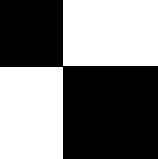Original                                                                   Image after filtering with kernel k

For example, a filter might be:

k = [9 6 4; 5 8 3; 2 4 9];

This filter representation is known as a convolution kernel. The MATLAB function conv2 implements image filtering by applying your convolution kernel to an image matrix. conv2 takes as arguments an input image and a filter, and returns an output image. For example, in this call, k is the convolution kernel, A is the input image, and B is the output image.

Another function which can be used is the imfilter function.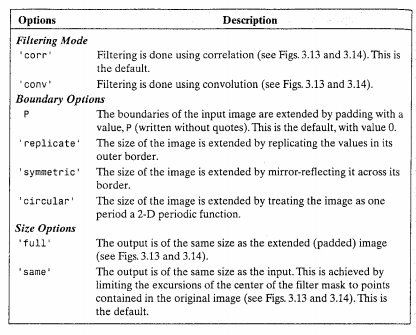#### Non-Linear FIltering

In addition to convolution, there are many other filtering operations you can
implement through sliding neighborhoods. Many of these operations are
nonlinear in nature. For example, you can implement a sliding neighborhood
operation where the value of an output pixel is equal to the standard deviation
of the values of the pixels in the input pixel’s neighborhood.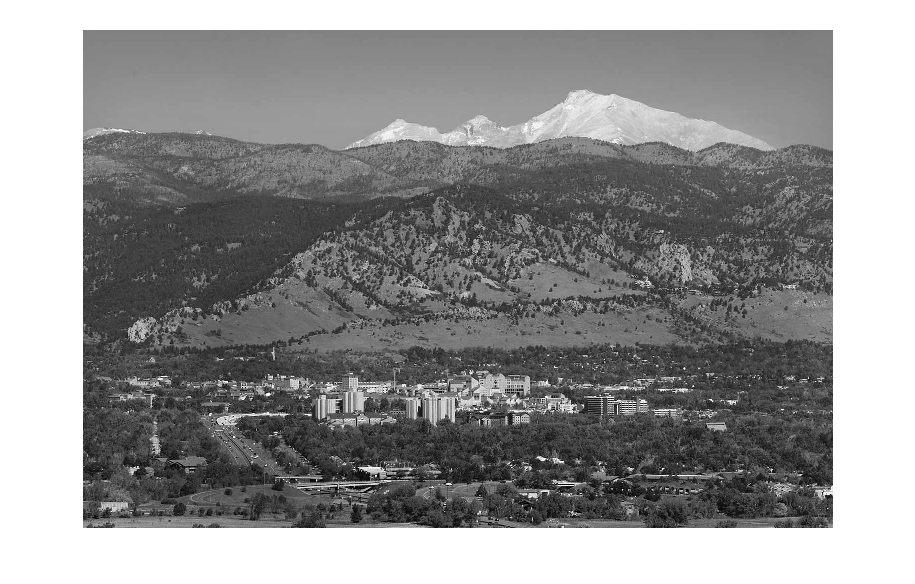Original Image

You can use the nlfilter function to implement a variety of sliding
neighborhood operations.nlfilter takes as input arguments an image, a
neighborhood size, and a function that returns a scalar, and returns an image
of the same size as the input image. The value of each pixel in the output image
is computed by passing the corresponding input pixel’s neighborhood to the
function. For example, this call computes each output pixel by taking the
standard deviation of the values of the input pixel’s 3-by-3 neighborhood (that
is, the pixel itself and its eight contiguous neighbors):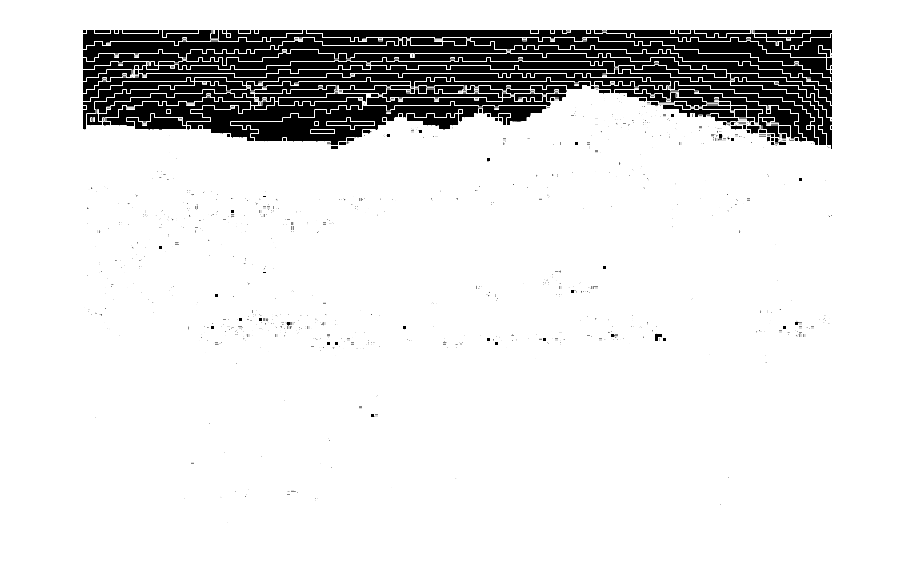Using ‘std2’

You can write an M-file to implement a specific function, and then use this
function with nlfilter. For example, this command processes the matrix I in
2-by-3 neighborhoods with a function called myfun.m:

You can also use an inline function; in this case, the function name appears in
the nlfilter call without quotation marks. For example,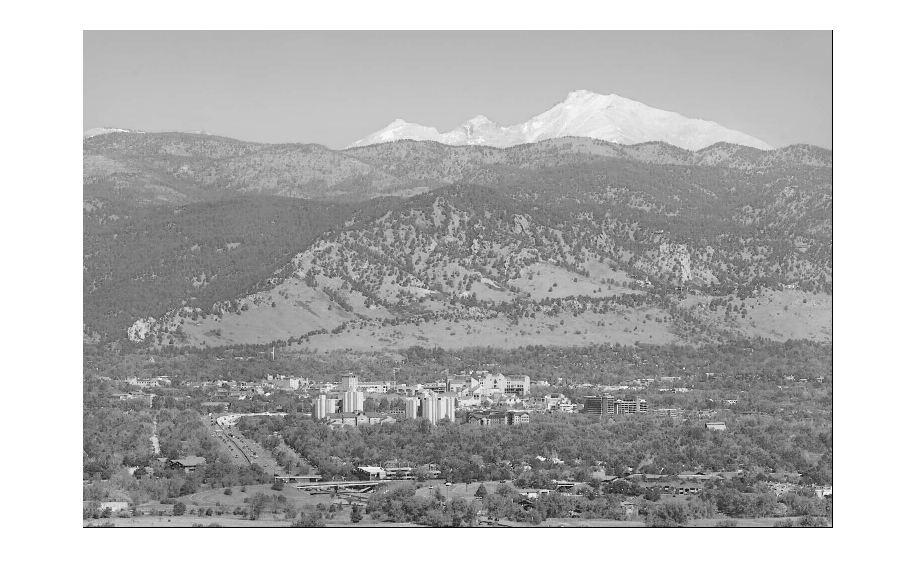Result

The example below uses nlfilter to set each pixel to the maximum value in
its 3-by-3 neighborhood.Result

The result in case of the previous command is quite apparent as some level of noise has appeared in the image.